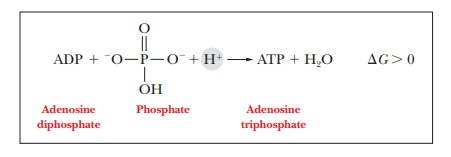Home | | Biochemistry | Spontaneity in Biochemical Reactions

# Spontaneity in Biochemical Reactions

How can we predict what reactions will happen in living cells?

Spontaneity in Biochemical Reactions

## How can we predict what reactions will happen in living cells?

The most useful criterion for predicting the spontaneity of a process is the freeenergy, which is indicated by the symbol G.(Strictly speaking, the use of thiscriterion requires conditions of constant temperature and pressure, which are usual in biochemical thermodynamics.) It is not possible to measure absolute values of energy; only the changes in energy that occur during a process can be measured. The value of the change in free energy, G (where the symbol indicates change), gives the needed information about the spontaneity of the process under consideration.

The free energy of a system decreases in a spontaneous (energy-releasing) process, so G is negative (G < 0). Such a process is called exergonic, mean-ing that energy is released. When the change in free energy is positive (G >0), the process is nonspontaneous. For a nonspontaneous process to occur, energy must be supplied. Nonspontaneous processes are also called ender-gonic, meaning that energy is absorbed. For a process at equilibrium, with nonet change in either direction, the change in free energy is zero (G= 0).

The sign of the change in free energy, G, indicates the direction of the reaction:An example of a spontaneous process is the aerobic metabolism of glucose, in which glucose reacts with oxygen to produce carbon dioxide, water, and energy for the organism.

Glucose + 6O2 6CO2 + 6H2O                                  G <0

An example of a nonspontaneous process is the reverse of the reaction that we saw  , the phosphorylation of ADP (adenosine diphos-phate) to give ATP (adenosine triphosphate). This reaction takes place in liv-ing organisms because metabolic processes supply energy.## Summary

The change in free energy (G) that accompanies a reaction deter-mines whether that reaction is spontaneous at a given temperature and pressure.

A negative free energy change (G< 0) is characteristic of a spontaneous reaction. A positive free energy change (G> 0) indicates that the reac-tion is not spontaneous, but the reverse process is spontaneous. When the free energy change is zero (G = 0), the reaction is at equilibrium.

Study Material, Lecturing Notes, Assignment, Reference, Wiki description explanation, brief detail
Biochemistry: Biochemistry and the Organization of Cells : Spontaneity in Biochemical Reactions |